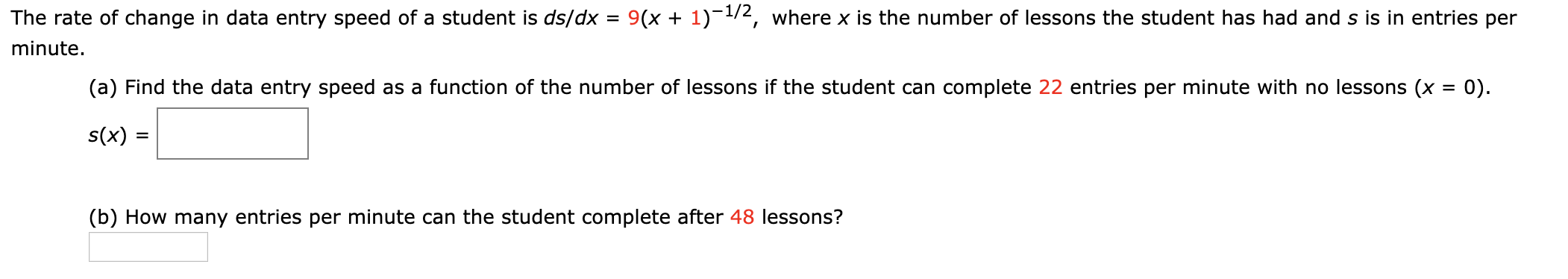# The rate of change in data entry speed of a student is ds/dx = 9(x + 1)-1/2, where x is the number of lessons the student has had and s is in entries perminute.(a) Find the data entry speed as a function of the number of lessons if the student can complete 22 entries per minute with no lessons (x = 0).s(x) :(b) How many entries per minute can the student complete after 48 lessons?

Question
16 viewshelp_outlineImage TranscriptioncloseThe rate of change in data entry speed of a student is ds/dx = 9(x + 1)-1/2, where x is the number of lessons the student has had and s is in entries per minute. (a) Find the data entry speed as a function of the number of lessons if the student can complete 22 entries per minute with no lessons (x = 0). s(x) : (b) How many entries per minute can the student complete after 48 lessons? fullscreen
check_circle

Step 1

To determine

Part a) The data entry speed as a function of the number of lessons the student has taken.

Part b) The number of entries per minute the student can complete after 48 lessons.

Step 2

Given:

The rate of change in data entry speed of a student is,

Step 3

Part (a)

Integrate the rate of change in data...

### Want to see the full answer?

See Solution

#### Want to see this answer and more?

Solutions are written by subject experts who are available 24/7. Questions are typically answered within 1 hour.*

See Solution
*Response times may vary by subject and question.
Tagged in